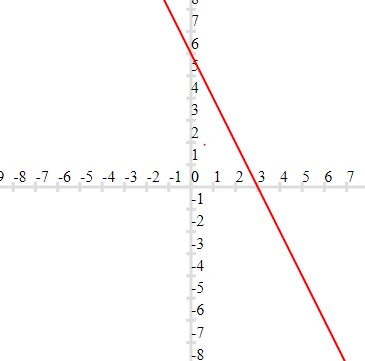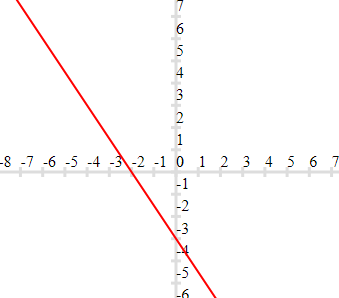# Linear Equations in two Variable Class 9 Notes

Welcome to Class 9 Maths chapter 4 Linear Equations in two Variable notes.The topics in this page are What is Linear equations, Linear equations Solutions and Graphical Representation of Linear equation in one and two variables along with examples, and questions and answers .This is according to CBSE and the NCERT textbook. If you like the study material, feel free to share the link as much as possible.

Table of Content

## What is Linear equations

• A linear equation is an algebraic equation in which each term is either a constant or the product of a constant and (the first power of) a single variable. Linear equations can have one or more variables
Example
$ax+b=0$, $a \neq 0$
a and b are constant
$ax+by+c=0$ , $a \neq 0$ and $b \neq 0$
a,b,c are constants
• In Linear equation ,No variable is raised to a power greater than 1 or used as the denominator of a fraction.
So, $ax^2 +b=0$ is not a linear equation
Similary $\frac {a}{x}+b=0$ is not a linear equation
• Linear equations  are  straight lines when plotted on Cartesian plane

## Linear equations Solutions

 S.no Type of equation Mathematical representation Solutions 1 Linear equation in one Variable $ax+b=0 \; , \; a \neq 0$ a and b are real number One solution 2 Linear equation in two Variables $ax+by+c=0 \; , \; a \neq 0 \; and \; b \neq 0$ i,e a and b both should not be zero. This condition is often represented as $a^2 + b^2 \ne 0$ a, b and c are real number Infinite solution possible 3 Linear equation in three Variables $ax+by+cz+d=0 \; , \; a \neq 0 \;, \; b \neq 0 \; and \; c \neq 0$ i,e a , b and c all should not be zero. This condition is often represented as $a^2 + b^2 +c^2 \ne 0$ a, b, c, d  are real number Infinite solution possible

Question 1.
Find four different solutions of the equation $x + 2y = 6$.
Solution
We can get many solutions of the linear equation by just picking a value of your choice for x
Here We can find the solutions by putting x=0,1,2,3
For x= 0
$0 +2y=6$ or $2y=6$ or y=3
So (0,3) is a solution
For x= 1
$1 +2y=6$ or $2y=5$ or y=2.5
So (1,2.5) is a solution
For x= 2
$2 +2y=6$ or $2y=4$ or y=2
So (2,2) is a solution
For x= 3
$3 +2y=6$ or $2y=3$ or y=1.5
So (3,1.5) is a solution
Hence the four solutions are
(0,3) , (1,2.5) ,(2,2) and (3,1.5)

## Graphical Representation of Linear equation in one and two variables

• Linear equation in two variables is represented by straight line the Cartesian plane.
• Every point on the line is the solution of the equation.
• Infact Linear equation in one variable can also be represented on Cartesian plane, it will be a straight line either parallel to x –axis or y –axis
$x-2=0$ , (straight line parallel to y axis). It means ( 2,<any value on y axis ) will satisfy this line
$y-2=0$, ( straight line parallel to x axis ). It means ( <any value on x-axis ),2 ) will satisfy this line

## Steps to Draw the Given line on Cartesian plane| how to graph the linear equations

1. Suppose the equation given is
$ax+by+c=0 \; , \; a \neq 0 \; and \; b \neq 0$
2. Find the value of y for x=0
$y=\frac {-c}{b}$
This point will lie on Y –axis. And the coordinates will be $(0,\frac{-c}{b})$
3. Find the value of x for y=0
$x=\frac {-c}{a}$
This point will lie on X –axis. And the coordinates will be $(\frac {-c}{a}, 0)$
4. Now we can draw the line joining these two points
5. It is easy to draw the points if the values are integers,So if you don't get integer in previous step you may choose point where the values are integers
Question 1.
Draw the graph of each of the equations given below. Also, find the co-ordinates of the point where the graph cuts the co-ordinates axis:
(a) 2x + y = 6
(b) 3x + 2y + 6 = 0
Solution
To draw the graph, we need at least two solutions of the equation We can get two points by putting x=0 and y=0. And then draw the graph. Corrdinates will be given by (x,0) and (0,y)

(a)Coordinates are (3,0) and (0,6)

(b)Coordinates are (-2,0) and (0,-3)

Question 2.
Any point on the y-axis is of the form
(a) (x, 0)
(b) (x, y)
(c) (0, y)
(d) ( y, y)
Solution

Question 3.
At what point does the graph of the linear equation x + y = 5 meet a line which is parallel to the y-axis, at a distance 2 units from the origin and in the positive direction of x-axis.
Solution
The coordinates of the points lying on the line parallel to the y-axis, at a distance 2 units from the origin and in the positive direction of the x-axis are of the form (2, a).
Putting x = 2, y = a in the equation x + y = 5, we get a = 3. Thus, the required point is (2, 3).